# Math Blocks

## number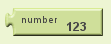Specifies a numeric value.

## greater than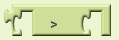Compares two given numbers. If the first is larger, returns true; otherwise, returns false.

## less than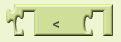Compares two given numbers. If the first is smaller, returns true; otherwise, returns false.

## less than or equal to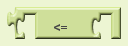Compares two given numbers. If the first is smaller than or equal to the second, returns true; otherwise, returns false.

## greater than or equal to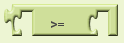Compares two given numbers. If the first is greater than or equal to the second, returns true; otherwise, returns false.

## equals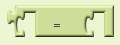Tests whether two given values are equal. If so, returns true; otherwise, returns false. This is the same as the block in the logic drawer and is repeated here for convenience. The arguments can be anything, not just numbers.

## +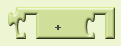Returns the sum of two given numbers.

## ?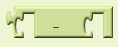Returns the result of subtracting the second number from the first.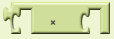Returns the product of two given numbers.

## /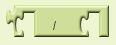Returns the result of dividing the first number by the second. For example, 1 divided by 3 is 0.3333.

## sqrt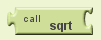Returns the square root of the given number.

## random fraction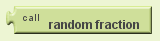Returns a random value between 0 and 1.

## random integerReturns a random integer value between the given values, inclusive. The order of the arguments doesn't matter.

## random set seed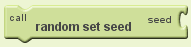Use this block to generate repeatable sequences of random numbers. You can generate the same sequence of random numbers by first calling random set seed with the same value. This is useful for testing programs that involve random values.

## negate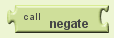Returns the negative of the given number.

## min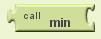Returns the smallest of a given set of numbers.

## max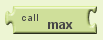Returns the largest of a given set of numbers.

## quotientReturns the result of dividing the first number by the second and discarding any fractional part of the result.

## remainderRemainder(a,b) returns the result of dividing a by b and taking the remainder. For example, remainder(11,5) = 1, remainder(-11, 5) = -1, remainder(11, -5) = 1, and remainder(-11, -5) = -1.

## modulo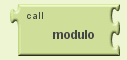Modulo(a,b) is the same as remainder(a,b) when a and b are positive. More generally, modulo(a,b) is defined for any a and b so that (floor(a/b) b) + modulo(a,b) = a. For example, modulo(11, 5) = 1, modulo(-11, 5) = 4, modulo(11, -5) = -4, modulo(-11, -5) = -1. Modulo(a,b) always has the same sign as b, while remainder(a,b) always has the same sign as a.

## abs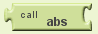Returns the absolute value of the given number.

## round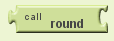Rounds the given number to the nearest integer and returns the result. If the number is midway between two integers, rounds to the even integer.

## floor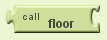Calculates the greatest integer that's less than or equal to the given number.

## ceiling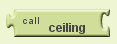Returns the smallest integer that's greater than or equal to the given number.

## exptRaises the first given number to the power of the second and returns the result.

## exp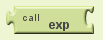Returns e (2.71828...) raised to the power of the given number and returns the result.

## log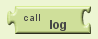Returns the natural logarithm of the given number.

## sin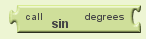Returns the sine of the given number in degrees.

## cos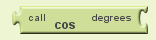Returns the cosine of the given number in degrees.

## tan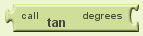Returns the tangent of the given number in degrees.

## asin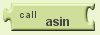Returns the arcsine of the given number in degrees.

## acos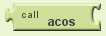Returns the arccosine of the given number in degrees.

## atan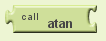Returns the arctangent of the given number in degrees.

## atan2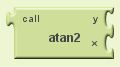Returns the arctangent of y/x, given y and x.

## format as decimal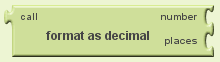Format a number as a decimal with a given number of places after the decimal point. The number of places must be a non-negative integer. The result is produced by rounding the number (if there were too many places) or by adding zeros on the right (if there were too few).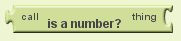Returns true if the given object is a number, and false otherwise.